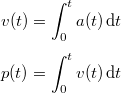Posts in category physics

# Damping with delta-time

Just a quick tip on how to convert usual damping code to something framerate-independent.

Most of us have probably, at some point, written code resembling this:

// Perform velocity damping
velocity -= velocity * 0.01f;


… or probably the more correct:

// Per-second damping coefficient
float const D = 10.0f;

// Damp velocity according to timestep
velocity -= velocity * D * delta_time;


Yet this is not fully framerate-independent; results are slightly different at 30fps and 60fps, and more importantly, spikes in the framerate cause lots of weird artifacts, causing developers to attempt to fix the situation by clamping delta_time, which is not ideal.

## The exponentiation method

Here is one way to fix it: assume that the code works correctly at 60 fps. This means that each frame, velocity is effectively multiplied by 1 - D / 60.

After one second, i.e. 60 frames, velocity has been multiplied by (1 - D / 60) ^ 60.

After two seconds, it has been multiplied by (1 - D / 60) ^ (60 * 2).

After N seconds, it has been multiplied by (1 - D / 60) ^ (60 * N).

So, there, we have a formula that tells us what happens after N seconds, and it’s a continuous function. We can therefore choose N as we like, and especially N = delta_time:

// Per-second damping coefficient
float const D = 10.0f;

// Damp velocity (framerate-independent)
velocity *= pow(1.f - D / 60.f, 60.f * delta_time);


Which can be conveniently rewritten as:

// Per-second damping coefficient
float const D = 10.0f;
// Exponentiation base for velocity damping
float const D2 = pow(1.f - D / 60.f, 60.f);

// Damp velocity (framerate-independent)
velocity *= pow(D2, delta_time);


## Use with lerp

The same method can be adapted to uses of linear interpolation such as this one:

// Perform velocity damping
velocity = lerp(velocity, target_velocity, K * delta_time);


Which we replace with:

// Damp velocity (framerate-independent)
velocity = lerp(velocity, target_velocity,
1.f - pow(1.f - K / 60.f, 60.f * delta_time));


# Understanding basic motion calculations in games: Euler vs. Verlet

During the past month, I have found myself in the position of having to explain the contents of this article to six different persons, either at work or over the Internet. Though there are a lot of articles on the subject, it’s still as if almost everyone gets it wrong. I was still polishing this article when I had the opportunity to explain it a seventh time.

And two days ago a coworker told me the source code of a certain framework disagreed with me… The kind of framework that probably has three NDAs preventing me from even thinking about it.

Well that framework got it wrong, too. So now I’m mad at the entire world for no rational reason other than the ever occurring realisation of the amount of wrong out there, and this article is but a catharsis to deal with my uncontrollable rage.

## A simple example

Imagine a particle with position Pos and velocity Vel affected by acceleration Accel. Let’s say for the moment that the acceleration is constant. This is the case when only gravity is present.

A typical game engine loop will update position with regards to a timestep (often the duration of a frame) using the following method, known as Euler integration:

Particle::Update(float dt)
{
Accel = vec3(0, 0, -9.81); /* Constant acceleration: gravity */
Vel = Vel + Accel * dt;    /* New, timestep-corrected velocity */
Pos = Pos + Vel * dt;      /* New, timestep-corrected position */
}


This comes directly from the definition of acceleration:Putting these two differential equations into Euler integration gives us the above code.

## Measuring accuracy

Typical particle trajectories would look a bit like this:These are three runs of the above simulation with the same initial values.

• once with maximum accuracy,
• once at 60 frames per second,
• once at 30 frames per second.

You can notice the slight inaccuracy in the trajectories.

You may think…“Oh, it could be worse; it’s just the expected inaccuracy with different framerate values.”

Well, no.

Just… no.If you are updating positions this way and you do not have a really good reason for doing so then either you or the person who taught you is a fucking idiot and should not have been allowed to write so-called physics code in the first place and I most certainly hope to humbly bestow enlightenment upon you in the form of a massive cluebat and don’t you dare stop reading this sentence before I’m finished.

## Why this is wrong

When doing kinematics, computing position from acceleration is an integration process. First you integrate acceleration with respect to time to get velocity, then you integrate velocity to get position.The integral of a function can be seen as the area below its curve. So, how do you properly get the integral of our velocity between t and t + dt, ie. the green area below?It’s not by doing new_velocity * dt (left image).

It’s not by doing old_velocity * dt either (middle image).

It’s obviously by doing (old_velocity + new_velocity) * 0.5 * dt (right image).

## And now for the correct code

This is what the update method should look like. It’s called Velocity Verlet integration (not strictly the same as Verlet integration, but with a similar error order) and it always gives the perfect, exact position of the particle in the case of constant acceleration, even with the nastiest framerate you can think of. Even at two frames per second.

Particle::Update(float dt)
{
Accel = vec3(0, 0, -9.81);
vec3 OldVel = Vel;
Vel = Vel + Accel * dt;
Pos = Pos + (OldVel + Vel) * 0.5 * dt;
}


And the resulting trajectories at different framerates:“Oh wow thank you. But what if acceleration is not constant, like in real life?”